# B. No-go result forin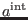We have seen in Appendix A that we can always take (193) in ( 181). Consequently, the first-order deformation of the solution to the master equation in the interacting case stops at antighost number four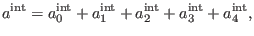(246)

where the components on the right-hand side of (194) are subject to the equations (68) and (66)-(67) for.

The pieceas solution to equation (68) forhas the general form expressed by (75) for, withfrom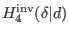. According to (81) at antighost number four, it follows thatis spanned by some representatives involving only BF generators. Sinceshould again mix the BF and the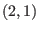tensor field sectors, it follows that one should retain from the basis elementsonly the objects containing at least one ghost from thetensor field sector, namelyor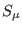. The general solution to (68) forreads as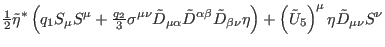(247)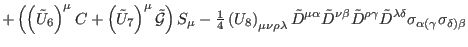(248)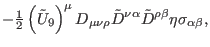(249)

where each element generically denoted byis the Hodge dual of an object similar to (83), but withreplaced by the arbitrary, smooth function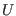, depending on the undifferentiated scalar field,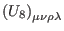reads as in (83) with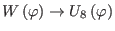, and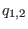are two arbitrary, real constants.

Introducing (195) in equation (66) forand using definitions (35)-(52), we determine the component of antighost number three fromin the form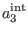(250)(251)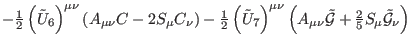(252)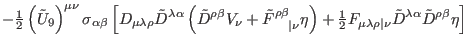(253)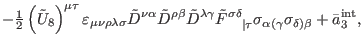(254)

where eachis the Hodge dual of an object of the type (84), withreplaced by the corresponding function of the type. Here,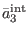is the general solution to the homogeneous equation (68) for, showing thatis a nontrivial object from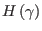in pure ghost number three.

At this point we decomposein a manner similar to (185)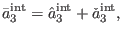(255)

where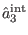is the solution to (68) forthat ensures the consistency of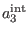in antighost number two, namely the existence of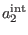as solution to (67 ) for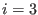with respect to the terms fromcontaining the functions of the typeor the constants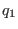or, while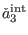is the solution to (68) forwhich is independently consistent in antighost number two(256)

Based on definitions (35)-(52) and taking into account decomposition (197), we get by direct computation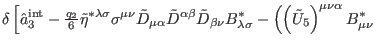(257)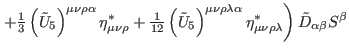(258)(259)(260)

where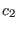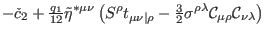(261)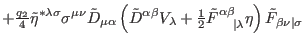(262)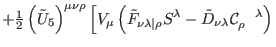(263)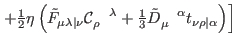(264)(265)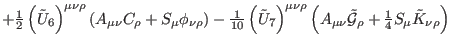(266)(267)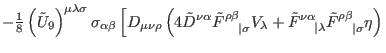(268)(269)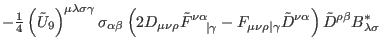(270)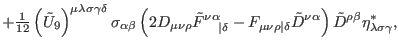(271)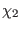(272)(273)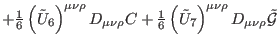(274)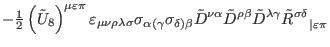(275)(276)

and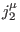are some local currents. Reprising an argument similar to that employed in Appendix A between equations (190) and (193), we find that the existence ofas solution to equation (67) forfinally implies thatexpressed by (201) must vanish. This is further equivalent to the fact that all the functions of the typemust be some real constants and both constantsmust vanish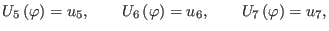(277)(278)

Inserting (202) and (203) in (195), we conclude that we can safely take(279)

in (194).

Ashkbiz Danehkar
2018-03-26